﻿Root Mean Square

# Root Mean Square

The root mean square (RootMeanSquare) (RMS) is defined as the square root of the mean square (the arithmetic mean of the squares of a set of numbers). The RMS is also known as the quadratic mean and is a particular case of the generalized mean with exponent 2. On the price charts it usually acts very similar to simple moving average indicator. But it can be very useful in case of charts with positive and negative values (e.g. z-score values).Calculation

In the case of a set of N values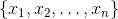, the RMS is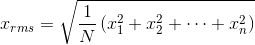.

If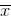is the arithmetic mean and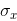is the standard deviation of a population then: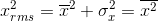Chart Example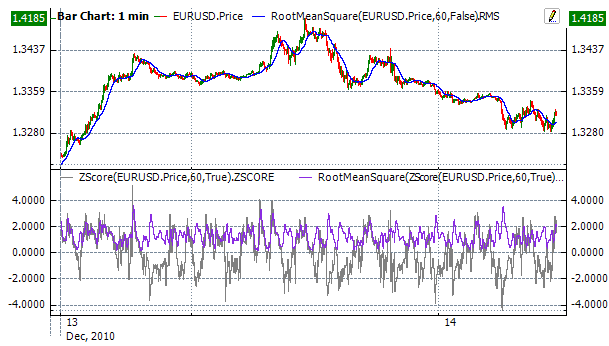Implementation and Usage

To initialize root mean square indicator, use one of the following constructors:

RootMeanSquare – set default values: period = 14

RootMeanSquare(Int32) – set value for period

RootMeanSquare(TimeSpan) – sets time period

Use

RMS - property to get current valueExample
C#
``` 1// Create new instance
2var indicator = new RootMeanSquare(28);
3
4// Number of stored values
5indicator.HistoryCapacity = 2;
6